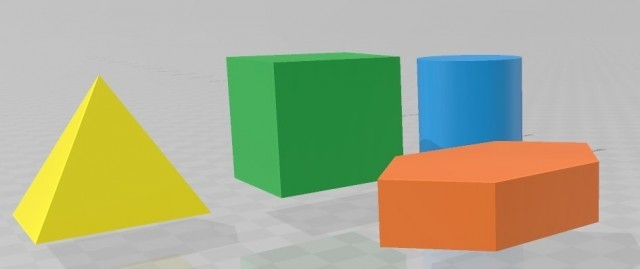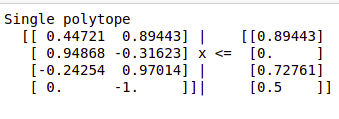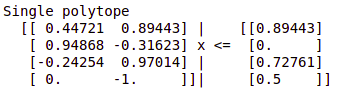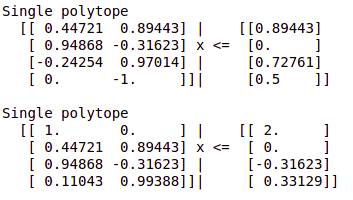Related Articles
Polytopes in Python
• Last Updated : 31 Dec, 2019

Python provides us with a third-party module to operate on polytopes. For one who doesn’t know what polytopes are, Polytopes are a geometrical form in an n-dimensional geometry corresponding to a polygon or polyhedron. Like other geometrical figures such as a square or a cube, a polytope isn’t limited to a single dimension. It can be 2-D, 3-D, or n-D.An n-dimensional polytope is generally represented as n-polytope. For example, a 2-D polytope will be represented as 2-polytope, a 3-D polytope as 3-polytope and so on. A polytope is stored inside Python using half-space representation or H-representation. This means that a polytope is represented as vector-matrix multiplication of two matrices and a vector:

`Ax <= B`

Here, A is an m*n matrix, x is a set of coordinates multiplied to A, and B is an m*1 column matrix. The polytope module will allow us to perform some geometrical operations on these polytopes. Let’s get started with the installation:

A polytope is a third-party module, so we would need to install it on our machine before we can use it. To install the module, just type this in the terminal:

`pip install polytope`

After installing, we will import the module into our IDE as:

`import polytope as pc`

We will now create a polytope using the polytope module:

 `# Python program to demonstrate``# polytopes`` ` ` ` `# Using numpy to create matrices``import` `numpy as np ``import` `polytope as pc`` ` ` ` `# Declaring numpy arrays``A ``=` `np.array([[``1.0``, ``2.0``],``              ``[``3.0``, ``-``1.0``],``              ``[``-``1.0``, ``4.0``],``              ``[``0.0``, ``-``2.0``]])`` ` `b ``=` `np.array([``2.0``, ``0.0``, ``3.0``, ``1.0``])`` ` `p ``=` `pc.Polytope(A, b)`` ` `print``(p)`

Output:Here, we created matrices A and b using numpy arrays. Then we used the `.Polytope()` function to create a polytope using those matrices. Here, we didn’t specify any value of “x”.

#### Methods and Operations on Polytopes:

Now that we have created a simple polytope, we can perform some basic operations on them. For example, the polytope module gives us the power to compare two polytopes.
Consider two polytopes, p1 and p2, then:

• p1 == p2: This means that every element of p1 is in p2 and every element of p2 is in p1. Hence, p1 and p2 are exactly identical.
• p1 <= p2: This means that p1 is a subset of p2. Therefore, every element of p1 is in p2 but the same may not be true for p2.
• p2 <= p1: This means that p2 is a subset of p1. Therefore, every element of p2 is in p1 but the same may not be true for p1.

We can also check if an element is in a polytope using:

`[4.0, 5.0] in p1`

Some basic mathematical operations available for polytopes include:

• p1.diff(p2)
• p1.union(p2)
• p1.intersect(p2)

For example:

 `# Python program to demonstrate``# polytopes`` ` ` ` `# Using numpy to create matrices``import` `numpy as np ``import` `polytope as pc `` ` `# Declaring numpy arrays``A ``=` `np.array([[``1.0``,  ``2.0``],``              ``[``3.0``, ``-``1.0``],``              ``[``-``1.0``, ``4.0``],``              ``[``0.0``, ``-``2.0``]])`` ` `b ``=` `np.array([``2.0``, ``0.0``, ``3.0``, ``1.0``])`` ` `C ``=` `np.array([[``1.0``,  ``0.0``],``              ``[``2.0``,  ``4.0``],``              ``[``3.0``, ``-``1.0``],``              ``[``1.0``,  ``9.0``]]) `` ` `d ``=` `np.array([``2.0``, ``0.0``, ``-``1.0``, ``3.0``])`` ` `p1 ``=` `pc.Polytope(A, b)``p2 ``=` `pc.Polytope(C, d)`` ` `# Using diff method``p1.diff(p2)`

Output:We can iterate over a region of polytopes. A region can be understood as a container containing two polytopes, where one is the beginning and the other is the end. Consider the following code.

 `# Python program to demonstrate``# polytopes`` ` ` ` `import` `numpy as np ``import` `polytope as pc `` ` ` ` `# Declaring numpy arrays``A ``=` `np.array([[``1.0``,  ``2.0``],``              ``[``3.0``, ``-``1.0``],``              ``[``-``1.0``, ``4.0``],``              ``[``0.0``, ``-``2.0``]])`` ` `b ``=` `np.array([``2.0``, ``0.0``, ``3.0``, ``1.0``])`` ` `C ``=` `np.array([[``1.0``,  ``0.0``],``              ``[``2.0``,  ``4.0``],``              ``[``3.0``, ``-``1.0``],``              ``[``1.0``,  ``9.0``]]) `` ` `d ``=` `np.array([``2.0``, ``0.0``, ``-``1.0``, ``3.0``])`` ` `p1 ``=` `pc.Polytope(A, b)``p2 ``=` `pc.Polytope(C, d)``r ``=` `pc.Region([p1, p2])`

The above code will create a region of two polytopes. This can be used as an iterator as:

 `for` `polytope ``in` `r:``    ``print``(polytope)`

output:Attention geek! Strengthen your foundations with the Python Programming Foundation Course and learn the basics.

To begin with, your interview preparations Enhance your Data Structures concepts with the Python DS Course. And to begin with your Machine Learning Journey, join the Machine Learning – Basic Level Course

My Personal Notes arrow_drop_up